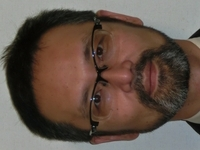## 【Staff】KUSAKARI Keiichirou

 KUSAKARI KeiichirouTitle Professor Department of Electrical, Electronic and Computer Engineering Informatics Course

#### Research fields

With drastic advancements of a computer in recent years, we can perform now easily "calculation" that cannot be done by humans. On the other hand, it is still difficult for a computer to give "mathematical proofs" that can be easily done by humans. This is because not only simple calculating ability but a certain sensitivity is required, in order to construct "mathematical proofs". Our laboratory researches automatic theorem proving that can reduce "mathematical proofs" to "calculation". As an application, we research program verification by an analogy with proofs in mathematics. As a base for researches above, we research computation model such as term rewriting system, lambda calculus, and so on.

#### Research Keywords

Program Theory, Term Rewriting System, Automatic Theorem Proving, Program Verification